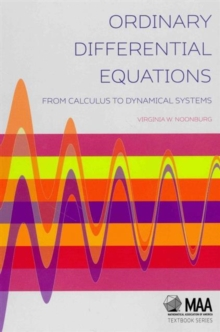# Ordinary Differential Equations : From Calculus to Dynamical Systems Hardback

## Part of the Mathematical Association of America Textbooks series

Hardback

• Information

#### Description

Techniques for studying ordinary differential equations (ODEs) have become part of the required toolkit for students in the applied sciences.

This book presents a modern treatment of the material found in a first undergraduate course in ODEs.

Standard analytical methods for first- and second-order equations are covered first, followed by numerical and graphical methods, and bifurcation theory.

Higher dimensional theory follows next via a study of linear systems of first-order equations, including background material in matrix algebra.

A phase plane analysis of two-dimensional nonlinear systems is a highlight, while an introduction to dynamical systems and an extension of bifurcation theory to cover systems of equations will be of particular interest to biologists.

With an emphasis on real-world problems, this book is an ideal basis for an undergraduate course in engineering and applied sciences such as biology, or as a refresher for beginning graduate students in these areas.

#### Information

• Format:Hardback
• Pages:326 pages
• Publisher:Mathematical Association of America
• Publication Date:
• Category:
• ISBN:9781939512048

£36.99

£32.34

£45.00

£38.83

£38.99

£32.36

£105.00

£84.90

£41.95

£36.50